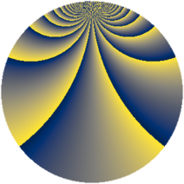# Properties

 Label 2240.2.duLevel $2240$ Weight $2$ Character orbit 2240.du Rep. character $\chi_{2240}(13,\cdot)$ Character field $\Q(\zeta_{16})$ Dimension $3040$ Sturm bound $768$

# Related objects

## Defining parameters

 Level: $$N$$ $$=$$ $$2240 = 2^{6} \cdot 5 \cdot 7$$ Weight: $$k$$ $$=$$ $$2$$ Character orbit: $$[\chi]$$ $$=$$ 2240.du (of order $$16$$ and degree $$8$$) Character conductor: $$\operatorname{cond}(\chi)$$ $$=$$ $$2240$$ Character field: $$\Q(\zeta_{16})$$ Sturm bound: $$768$$

## Dimensions

The following table gives the dimensions of various subspaces of $$M_{2}(2240, [\chi])$$.

Total New Old
Modular forms 3104 3104 0
Cusp forms 3040 3040 0
Eisenstein series 64 64 0

## Trace form

 $$3040q - 16q^{2} - 8q^{7} - 16q^{8} + O(q^{10})$$ $$3040q - 16q^{2} - 8q^{7} - 16q^{8} - 32q^{11} + 32q^{14} - 16q^{15} - 32q^{16} - 16q^{18} - 16q^{21} - 16q^{22} - 16q^{23} - 16q^{25} - 8q^{28} + 96q^{30} - 16q^{32} - 8q^{35} - 32q^{36} - 16q^{37} - 8q^{42} - 16q^{43} - 32q^{46} - 16q^{50} - 32q^{51} - 16q^{53} - 16q^{56} - 16q^{57} - 144q^{58} - 16q^{60} - 16q^{63} - 32q^{65} - 16q^{67} - 8q^{70} + 96q^{71} - 16q^{72} - 8q^{77} + 288q^{78} - 32q^{81} + 64q^{85} - 32q^{86} + 160q^{88} - 16q^{91} + 128q^{92} - 64q^{93} - 16q^{98} + O(q^{100})$$

## Decomposition of $$S_{2}^{\mathrm{new}}(2240, [\chi])$$ into newform subspaces

The newforms in this space have not yet been added to the LMFDB.